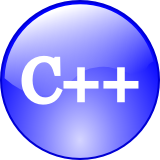# C/C++ Functions to Convert to UPPER CASE and lower caseC and C++ implementations that follow the standard library provide two functions in the header ctype.h to convert to upper and lower cases.

```char upperA = toupper('x');
char lowerA = tolower('X');
```

But if you want to write your own function to convert cases, here are two functions that use the string.h header.

## C and C++ Functions to Convert to lower case

```string lowercase(string s)
{
for (unsigned int i = 0; i < s.size(); i++)
if (s[i] >= 0x41 && s[i] <= 0x5A)
s[i] = s[i] + 0x20;
return s;
}
```

## C and C++ Functions to Convert to UPPER CASE

```string uppercase(string s)
{
for (unsigned int i = 0; i < s.size(); i++)
if (s[i] >= 0x61 && s[i] <= 0x7A)
s[i] = s[i] - 0x20;
return s;
}
```